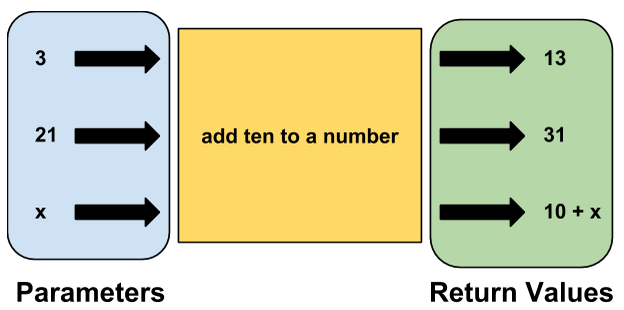# Methods and Return Values

Similar to how methods can take in values as parameters, methods can also return values.

## Keeping Results

Recall the `addTen` method from the previous chapter:

``````public void addTen(int x)
{
int xPlusTen = x + 10;
System.out.println(xPlusTen);
}
``````This method takes in a parameter, `x`, and adds 10 to it. Lastly, the program prints the value to the console.

But what if we wanted to store the value of `x + 10`? In the method above, `x + 10` is stored into a local variable called `xPlusTen`. However, this variable is lost when the method is finished -- it cannot be accessed outside of the method.

Using a return statement will allow us to pass a value back out of the method. That return value can be stored into a variable for later use.

Here's how to rewrite `addTen` to return a value instead of printing:

``````public int addTen(int x)
{
int xPlusTen = x + 10;
return xPlusTen;
}
``````

There are a few differences here. First, take a look at the first line of the method: `public int addTen(int x)`. Instead of `void`, we now use `int`. This tells the program that the `addTen` method will return a value that is an int. This is called the return type of the method. Up to this point, we've only used the `void` return type, which indicates that the method does not return anything. Because the `addTen` method will return an integer, we set the return type to `int`.

Note also that the `return` keyword does not require parentheses. Also, returning a value does not print that value to the console, similar to how passing in a value as a parameter does not print the value to the console.

## Calling a Method With a Return Value

A return value by itself would not be very useful, given that it does not print to the console. Fortunately, we can store a method's return value into a variable. Take a look at the following code:

``````int num = 7;
``````

First, we create a variable named `num` and initialize it to 7. Then, we create another variable called `tenAdded`. Notice that `tenAdded` is not given a normal value. Instead, we are setting it equal to `addTen(7)`. This means that the `tenAdded` variable will hold the result of whatever the function call `addTen(7)` returns. We know that `addTen(7)` will return 17, so `tenAdded` will be `17`.## Multiple Parameters With a Return Value

Return values work in many situations. For example, we can rewrite the `add` method from the previous section to return the sum instead of print it to the screen:

``````public int add(int x, int y)
{
int sum = x + y;
return sum;
}
``````

We can now call the method and store its return values

``````int sum = add(10, 90);
System.out.println(sum);
``````

which would print `100` to the console.